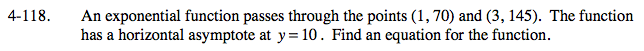### Home > PC > Chapter 4 > Lesson 4.2.4 > Problem4-118

4-118.Write the general equation for this problem.

Substitute the points in for (x, y), creating two equations and two unknowns.

Subtract 10 on both sides.
Then divide one equation by the other.

Substitute the b-value back into one of the original equations and solve for a. Then substitute a and b into the general equation.

y = a(bx) + 10

145 = a(b3) + 10
70 = a(b1) + 10

135 = a(b3)
60 = a(b1)
2.25 = b2
b = 1.5